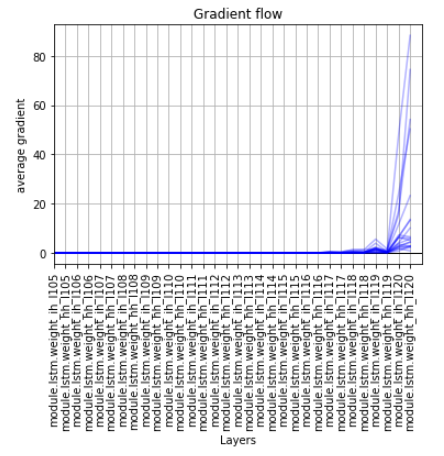# How can I optimize gradient flow in LSTM with Pytorch?

Hello!

I’m working in lstm with time-series data and I’ve observed a problem in the gradients of my network. I’ve one layer of 121 lstm cells. For each cell I’ve one input value and I get one output value. I work with a batch size of 121 values and I define lstm cell with batch_first = True, so my outputs are [batch,timestep,features].

Once I’ve the outputs (tensor of size [121,121,1]), I calculate the loss using MSELoss() and I backpropagate it. And here appears the problem. Looking the gradients of each cell, I notice that the gradients of first 100 cells (more or less) are null.

In theory, if I’m not wrong, when I backpropagate the error I calculate a gradient for each output, so I have a gradient for each cell. If that is true, I can’t understand why in the first cells they are zero.

Does somebody knows what is happening?

Thank you!

PS.: I show you the gradient flow of the last cells: